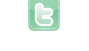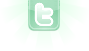## Trying to make a tank that faces whichever way its moving

I am trying to figure out how to make a game where you drive a tank and whenever you change directions from left to right and vice versa it will flip to go the direction you are now moving in. I am also going to try and make it a game where you try to hit objects and the way you shoot is similar to the game Bowmasters, if you know what that is. You can help with that if you want, which I would appreciate, but it is not what I need help with at the moment. I am able to make it change directions, but, I have the barrel as a separate object, and whenever I go from right to left, it is pointing at the same angle as it was before, but in the opposite direction. So if it was pointing up at a 35 degree angle from its original angle, it is now pointing down at a 35 degree angle. What do I need to change? This is my current code on the first barrel that there is when the program starts. I have only done up so far.
```int delay1=0;
int rotation=30;
public void act()
{
delay1++;
if (Greenfoot.isKeyDown("d"))
{
move(5);
}
if (Greenfoot.isKeyDown("up") && delay1>=rotation)
{
setRotation(getRotation()-1);
delay1=0;
if (getRotation() <=345)
{
setRotation(getRotation()+1);
}
}
if (Greenfoot.isKeyDown("a"))
{
BarrelLeft left = new BarrelLeft();
`left.setRotation(180-getRotation());`
`left.setRotation(270-getRotation());`